# Diagnostic methods for Building the regression model

29 Jun 2016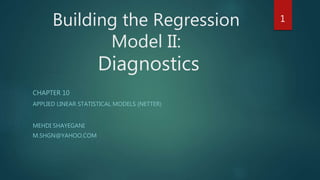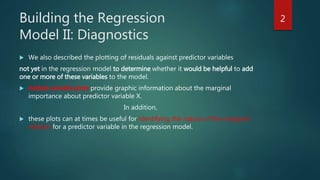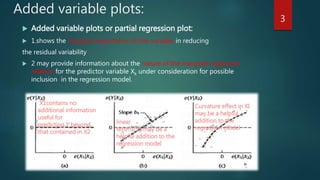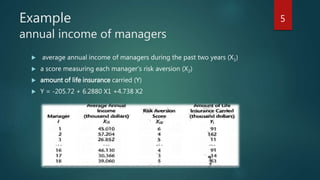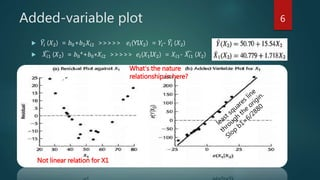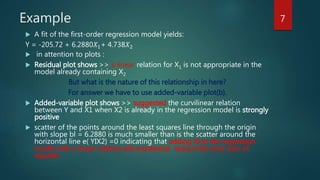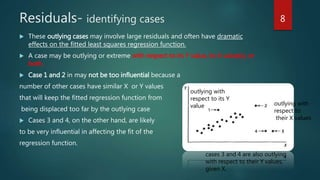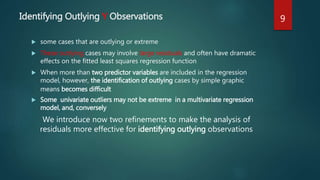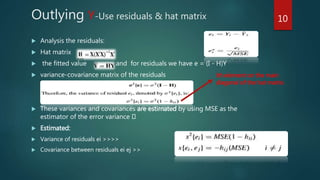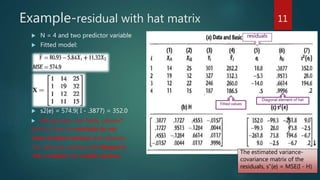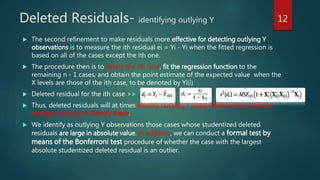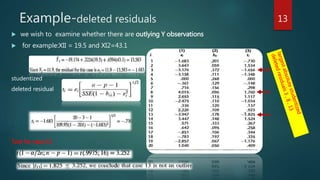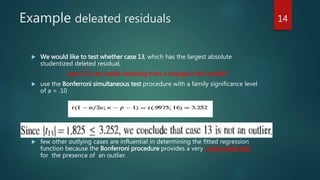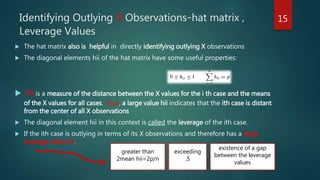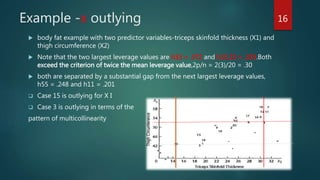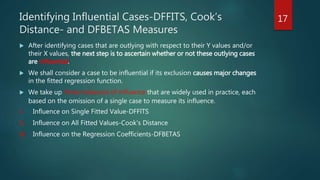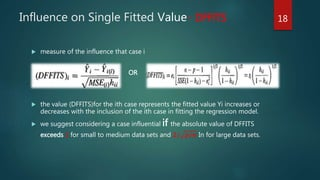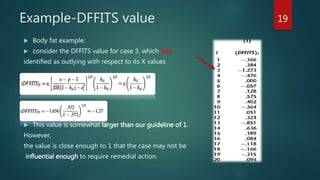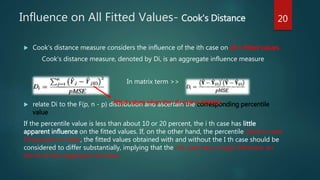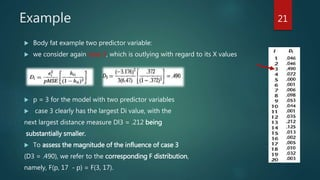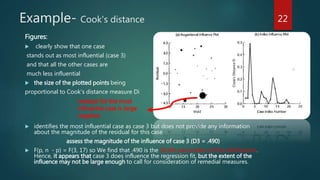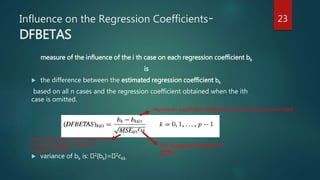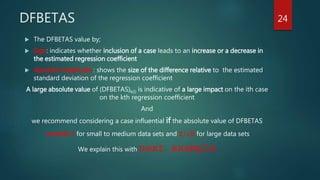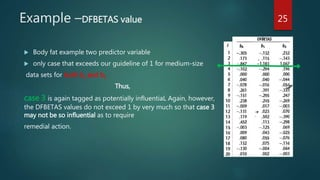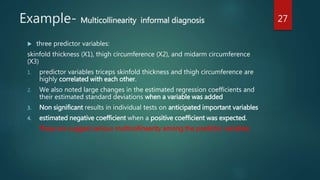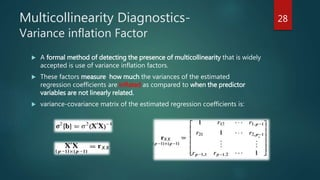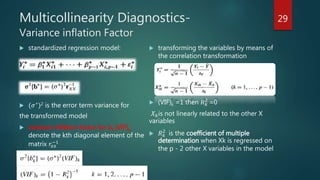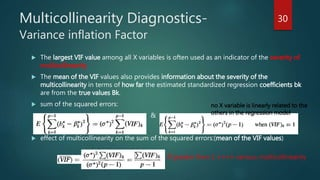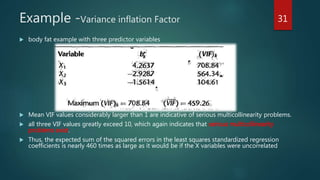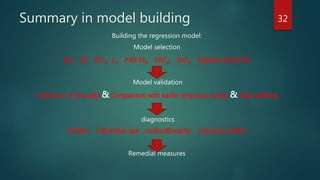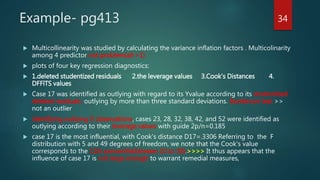1 sur 35

### Diagnostic methods for Building the regression model

• 1. Building the Regression Model II: Diagnostics CHAPTER 10 APPLIED LINEAR STATISTICAL MODELS (NETTER) MEHDI SHAYEGANI M.SHGN@YAHOO.COM 1
• 2. Building the Regression Model II: Diagnostics  We also described the plotting of residuals against predictor variables not yet in the regression model to determine whether it would be helpful to add one or more of these variables to the model.  Added-variable plots provide graphic information about the marginal importance about predictor variable X. In addition,  these plots can at times be useful for identifying the nature of the marginal relation for a predictor variable in the regression model. 2
• 3. Added variable plots:  Added variable plots or partial regression plot:  1.shows the marginal importance of this variable in reducing the residual variability  2 may provide information about the nature of the marginal regression relation for the predictor variable Xk under consideration for possible inclusion in the regression model. 3 X1contains no additional information useful for predicting Y beyond that contained in X2 linear term in XI may be a helpful addition to the regression model Curvature effect in XI may be a helpful addition to the regression model
• 4. Multiple regression model with two predictor variables X1 and X2  In the previous plots:  X2 is already in the regression model and X1 is under consideration to be added.  Plot A) X1 contains no additional information useful for predicting Y beyond that contained in X2  Plot B,C) addition of X1 to the regression model may be helpful and suggesting the possible nature of the curvature effect by the pattern shown. 4
• 5. Example annual income of managers  average annual income of managers during the past two years (X1)  a score measuring each manager's risk aversion (X2)  amount of life insurance carried (Y)  Y = -205.72 + 6.2880 X1 +4.738 X2 5
• 6. Added-variable plot  𝑌𝑖 (𝑋2) = 𝑏0+𝑏2 𝑋𝑖2 >>>>> 𝑒𝑖(YI𝑋2) = 𝑌𝑖- 𝑌𝑖 (𝑋2)  𝑋𝑖1 (𝑋2) = 𝑏0*+𝑏0∗𝑋𝑖2 >>>>> 𝑒𝑖(𝑋1I𝑋2) = 𝑋𝑖1- 𝑋𝑖1 (𝑋2) What's the nature relationship in here? Not linear relation for X1 6
• 7. Example  A fit of the first-order regression model yields: Y = -205.72 + 6.2880𝑋1+ 4.738𝑋2  in attention to plots :  Residual plot shows >> a linear relation for X1 is not appropriate in the model already containing X2 But what is the nature of this relationship in here? For answer we have to use added-variable plot(b).  Added-variable plot shows >> suggested the curvilinear relation between Y and X1 when X2 is already in the regression model is strongly positive  scatter of the points around the least squares line through the origin with slope bl = 6.2880 is much smaller than is the scatter around the horizontal line e( YIX2) =0 indicating that adding XI to the regression model with a linear relation will substantial reduce the error sum of squares 7
• 8. Residuals- identifying cases  These outlying cases may involve large residuals and often have dramatic effects on the fitted least squares regression function.  A case may be outlying or extreme with respect to its Y value, its X value(s), or both.  Case 1 and 2 in may not be too influential because a number of other cases have similar X or Y values that will keep the fitted regression function from being displaced too far by the outlying case  Cases 3 and 4, on the other hand, are likely to be very influential in affecting the fit of the regression function. 8 outlying with respect to its Y value outlying with respect to their X values cases 3 and 4 are also outlying with respect to their Y values, given X.
• 9. Identifying Outlying Y Observations  some cases that are outlying or extreme  These outlying cases may involve large residuals and often have dramatic effects on the fitted least squares regression function  When more than two predictor variables are included in the regression model, however, the identification of outlying cases by simple graphic means becomes difficult  Some univariate outliers may not be extreme in a multivariate regression model, and, conversely We introduce now two refinements to make the analysis of residuals more effective for identifying outlying observations 9
• 10. Outlying Y-Use residuals & hat matrix  Analysis the residuals:  Hat matrix  the fitted value and for residuals we have e = (I - H)Y  variance-covariance matrix of the residuals  These variances and covariances are estimated by using MSE as the estimator of the error variance  Estimated:  Variance of residuals ei >>>>  Covariance between residuals ei ej >> 10 ith element on the main diagonal of the hat matrix
• 11. Example-residual with hat matrix  N = 4 and two predictor variable  Fitted model:  s2{e} = 574.9( I - .3877) = 352.0  We see from last Table, column7 (s2{ ei }), that the residuals do not have constant variance and residuals for cases are outlying with Respect to the x variable have smaller variance. The estimated variance- covariance matrix of the residuals, s"{e} = MSE(I - H) 11 Fitted values residuals Diagonal element of hat
• 12. Deleted Residuals- identifying outlying Y  The second refinement to make residuals more effective for detecting outlying Y observations is to measure the ith residual ei = Yi - Yi when the fitted regression is based on all of the cases except the ith one.  The procedure then is to delete the ith case, fit the regression function to the remaining n - 1 cases, and obtain the point estimate of the expected value when the X levels are those of the ith case, to be denoted by Yi(i)  Deleted residual for the ith case >>  Thus, deleted residuals will at times identify outlying Y observations when ordinary residuals would not identify these.  We identify as outlying Y observations those cases whose studentized deleted residuals are large in absolute value. In addition, we can conduct a formal test by means of the Bonferroni test procedure of whether the case with the largest absolute studentized deleted residual is an outlier. 12
• 13. Example-deleted residuals  we wish to examine whether there are outlying Y observations  for example:XII = 19.5 and XI2=43.1 studentized deleted residual Test for case 13 13
• 14. Example deleated residuals  We would like to test whether case 13, which has the largest absolute studentized deleted residual, case 13 is an outlier resulting from a change in the model?  use the Bonferroni simultaneous test procedure with a family significance level of a = .10  few other outlying cases are influential in determining the fitted regression function because the Bonferroni procedure provides a very conservative test for the presence of an outlier. 14
• 15. Identifying Outlying X Observations-hat matrix , Leverage Values  The hat matrix also is helpful in directly identifying outlying X observations  The diagonal elements hii of the hat matrix have some useful properties:  hii is a measure of the distance between the X values for the i th case and the means of the X values for all cases. Thus, a large value hii indicates that the ith case is distant from the center of all X observations  The diagonal element hii in this context is called the leverage of the ith case.  If the ith case is outlying in terms of its X observations and therefore has a large leverage value hii. 15 greater than 2mean hii=2p/n exceeding .5 existence of a gap between the leverage values
• 16. Example -x outlying  body fat example with two predictor variables-triceps skinfold thickness (X1) and thigh circumference (X2)  Note that the two largest leverage values are h33 = .372 and h15.15 = .333.Both exceed the criterion of twice the mean leverage value,2p/n = 2(3)/20 = .30  both are separated by a substantial gap from the next largest leverage values, h55 = .248 and h11 = .201  Case 15 is outlying for X I  Case 3 is outlying in terms of the pattern of multicollinearity 16
• 17. Identifying Influential Cases-DFFITS, Cook’s Distance- and DFBETAS Measures  After identifying cases that are outlying with respect to their Y values and/or their X values, the next step is to ascertain whether or not these outlying cases are influential.  We shall consider a case to be influential if its exclusion causes major changes in the fitted regression function.  We take up three measures of influence that are widely used in practice, each based on the omission of a single case to measure its influence. I. Influence on Single Fitted Value-DFFlTS II. Influence on All Fitted Values-Cook's Distance III. Influence on the Regression Coefficients-DFBETAS 17
• 18. Influence on Single Fitted Value- DFFlTS  measure of the influence that case i OR  the value (DFFITS)for the ith case represents the fitted value Yi increases or decreases with the inclusion of the ith case in fitting the regression model.  we suggest considering a case influential if the absolute value of DFFITS exceeds 1 for small to medium data sets and 𝟐/ 𝒑/𝒏 In for large data sets. 18
• 19. Example-DFFITS value  Body fat example:  consider the DFFITS value for case 3, which was identified as outlying with respect to its X values  This value is somewhat larger than our guideline of 1. However, the value is close enough to 1 that the case may not be influential enough to require remedial action. 19
• 20. Influence on All Fitted Values- Cook's Distance  Cook's distance measure considers the influence of the ith case on all n fitted values. Cook's distance measure, denoted by Di, is an aggregate influence measure In matrix term >>  relate Di to the F(p, n - p) distribution and ascertain the corresponding percentile value If the percentile value is less than about 10 or 20 percent, the i th case has little apparent influence on the fitted values. If, on the other hand, the percentile value is near 50 percent or more, the fitted values obtained with and without the I th case should be considered to differ substantially, implying that the i th case has a major influence on the fit of the regression function. 20 fitted values when the i th case is deleted
• 21. Example  Body fat example two predictor variable:  we consider again case 3, which is outlying with regard to its X values  p = 3 for the model with two predictor variables  case 3 clearly has the largest Di value, with the next largest distance measure Dl3 = .212 being substantially smaller.  To assess the magnitude of the influence of case 3 (D3 = .490), we refer to the corresponding F distribution, namely, F(p, 17 - p) = F(3, 17). 21
• 22. Example- Cook's distance Figures:  clearly show that one case stands out as most influential (case 3) and that all the other cases are much less influential  the size of the plotted points being proportional to Cook's distance measure Di  identifies the most influential case as case 3 but does not provide any information about the magnitude of the residual for this case assess the magnitude of the influence of case 3 (D3 = .490)  F(p, n - p) = F(3, 17) so We find that .490 is the 30.6th percentiles of this distribution. Hence, it appears that case 3 does influence the regression fit, but the extent of the influence may not be large enough to call for consideration of remedial measures. 22 residual for the most influential case is large negative
• 23. Influence on the Regression Coefficients- DFBETAS measure of the influence of the i th case on each regression coefficient bk is  the difference between the estimated regression coefficient bk based on all n cases and the regression coefficient obtained when the ith case is omitted.  variance of bk is: 2{bk}= 2ckk kth diagonal element of (X'X)-1 regression coefficient obtained when the ith case is omitted error mean square obtained when the ith case is deleted in fitting the regression model 23
• 24. DFBETAS  The DFBETAS value by;  Sign: indicates whether inclusion of a case leads to an increase or a decrease in the estimated regression coefficient  absolute magnitude : shows the size of the difference relative to the estimated standard deviation of the regression coefficient A large absolute value of (DFBETAS)k(i) is indicative of a large impact on the ith case on the kth regression coefficient And we recommend considering a case influential if the absolute value of DFBETAS exceeds 1 for small to medium data sets and 𝟐/ 𝒏 for large data sets We explain this with next example 24
• 25. Example –DFBETAS value  Body fat example two predictor variable  only case that exceeds our guideline of 1 for medium-size data sets for both b1 and b2 Thus, case 3 is again tagged as potentially influentiaL Again, however, the DFBETAS values do not exceed 1 by very much so that case 3 may not be so influential as to require remedial action. 25
• 26. Multicollinearity Diagnostics- informal diagnostics  Indications of the presence of serious multicollinearity are given by the following informal diagnostics: 1. Large changes in the estimated regression coefficients when a predictor variable is added or deleted, or when an observation is altered or deleted 2. Non significant results in individual tests on the regression coefficients for important predictor variables. 3. Estimated regression coefficients with an algebraic sign that is the opposite of that expected from theoretical considerations or prior experience. 4. Large coefficients of simple correlation between pairs of predictor variables in the correlation matrix rxx. 5. Wide confidence intervals for the regression on coefficients representing important predictor variables. 26
• 27. Example- Multicollinearity informal diagnosis  three predictor variables: skinfold thickness (X1), thigh circumference (X2), and midarm circumference (X3) 1. predictor variables triceps skinfold thickness and thigh circumference are highly correlated with each other. 2. We also noted large changes in the estimated regression coefficients and their estimated standard deviations when a variable was added 3. Non significant results in individual tests on anticipated important variables 4. estimated negative coefficient when a positive coefficient was expected. These are suggest serious multicollinearity among the predictor variables 27
• 28. Multicollinearity Diagnostics- Variance inflation Factor  A formal method of detecting the presence of multicollinearity that is widely accepted is use of variance inflation factors.  These factors measure how much the variances of the estimated regression coefficients are inflated as compared to when the predictor variables are not linearly related.  variance-covariance matrix of the estimated regression coefficients is: 28
• 29. Multicollinearity Diagnostics- Variance inflation Factor  standardized regression model:  (𝜎∗)2 is the error term variance for the transformed model  variance inflation factor for bk (VIF)k denote the kth diagonal element of the matrix 𝑟𝑥𝑥 −1  transforming the variables by means of the correlation transformation  (VIF)k =1 then 𝑅 𝑘 2 =0 𝑋 𝑘is not linearly related to the other X variables  𝑅 𝑘 2 is the coefficient of multiple determination when Xk is regressed on the p - 2 other X variables in the model 29
• 30. Multicollinearity Diagnostics- Variance inflation Factor  The largest VIF value among all X variables is often used as an indicator of the severity of multicollinearity  The mean of the VIF values also provides information about the severity of the multicollinearity in terms of how far the estimated standardized regression coefficients bk are from the true values Bk.  sum of the squared errors: &  effect of multicollinearity on the sum of the squared errors:(mean of the VIF values) 30 no X variable is linearly related to the others in the regression model If greater then 1 >>>> serious multicollinearity
• 31. Example -Variance inflation Factor  body fat example with three predictor variables  Mean VIF values considerably larger than 1 are indicative of serious multicollinearity problems.  all three VIF values greatly exceed 10, which again indicates that serious multicollinearity problems exist.  Thus, the expected sum of the squared errors in the least squares standardized regression coefficients is nearly 460 times as large as it would be if the X variables were uncorrelated 31
• 32. Summary in model building Building the regression model: Model selection 𝑹 𝒂,𝑷 𝟐 𝑹 𝑷 𝟐 𝑨𝑰𝑪 𝒑 𝑪 𝒑 𝑷𝑹𝑬𝑺𝑺 𝒑 𝑺𝑩𝑪 𝒑 𝑺𝑺𝑬 𝒑 Stepwise Methods Model validation Collection of new data & Comparison with earlier empirical results & Data Splitting diagnostics Outliers influential case multicollinearity interaction effect Remedial measures 32
• 33. Example – surgical unit  Model selected : lny = 𝑋1 + 𝑋2 + 𝑋3 + 𝑋8  Examine the interaction effect with added variable plots for 6 two factor interaction:  To examine interaction effects further, a regression model containing first- order terms in XI, X2, X3, and X8 was fitted and added-variable plots for the six two-factor interaction terms.  these plots did not suggest that any strong two-variable interactions are present and need to be included in the model.  The residual plots shows no evidence of serious departures from the model.  use a residual plot and an added-variable plot to study graphically the strength of the marginal relationship between X5 and the response when X1, X2, X3, and X8 are already in the model. 33
• 34. Example- pg413  Multicollinearity was studied by calculating the variance inflation factors . Multicolinarity among 4 predictor not problem(all >1)  plots of four key regression diagnostics:  1.deleted studentized residuals 2.the leverage values 3.Cook's Distances 4. DFFlTS values  Case 17 was identified as outlying with regard to its Yvalue according to its studentized deleted residuals. outlying by more than three standard deviations. Bonferroni test >> not an outlier  identifying outlying X observations, cases 23, 28, 32, 38, 42, and 52 were identified as outlying according to their leverage values with guide 2p/n=0.185  case 17 is the most influential, with Cook's distance D17=.3306 Referring to the F distribution with 5 and 49 degrees of freedom, we note that the Cook's value corresponds to the 11th percentile(bitween 10 to 30).>>>> It thus appears that the influence of case 17 is not large enough to warrant remedial measures, 34
• 35. 35